# 1. Introduction

CancerSubtypes is a package for cancer subtype analysis that includes various functions from dataset processing to result validation. In CancerSubtypes package, we provide a unified framework for analysing cancer subtpes from raw data to result visualisation. The main functions include genomic data pre-processing, cancer subtypes identification, results validation, visualization and comparison. CancerSubtypes provides the common data imputation and normalization methods for the genomic data pre-processing. Meanwhile, there are four feature selection methods to screen the key features in genomic dataset. The common cancer subtypes identification methods are integrated in this package such as Consensus clustering (CC) [From R package ConsensusClusterPlus], Consensus Nonnegative matrix factorization (CNMF) [From R package NMF], Integrative clustering (iCluster)[From R package iCluster], Similarity Network Fusion (SNF) [From R package SNFtool], Combined SNF and CC (SNF.CC) and Weighted Similarity Network Fusion (WSNF). We implement these cancer subtypes identification methods in a unified input and output data format. The process of analysing cancer subtypes can be easily conducted in a standard workflow. CancerSubtypes provides the most useful feature selection methods and subtypes validation method and helps users to focus on their cancer genomic data and the results from different methods can be compared and evaluation in visualization way easily.

# 2 Data processing

For the basic data processing, CancerSubtypes provides the methods for data distribution check, imputation and normalization and feature selection. There are four feature selection methods (Variance-Var, Median Absolute Deviation-MAD, COX model, Principal Component Analysis-PCA) in CancerSubtypes package. All the data processing methods possess the same input and output data format.

### 2.1 Basic processing

#### 2.1.1 Analysis the raw data by check the data distribution.

``````### Prepare a TCGA gene expression dataset for analysis.
library(CancerSubtypes)
library("RTCGA.mRNA")
rm(list = ls())
data(BRCA.mRNA)
mRNA=t(as.matrix(BRCA.mRNA[,-1]))
colnames(mRNA)=BRCA.mRNA[,1]

###To observe the mean, variance and Median Absolute Deviation distribution of the dataset, it helps users to get the distribution characteristics of the data, e.g. To evaluate whether the dataset fits a normal distribution or not.
data.checkDistribution(mRNA)``````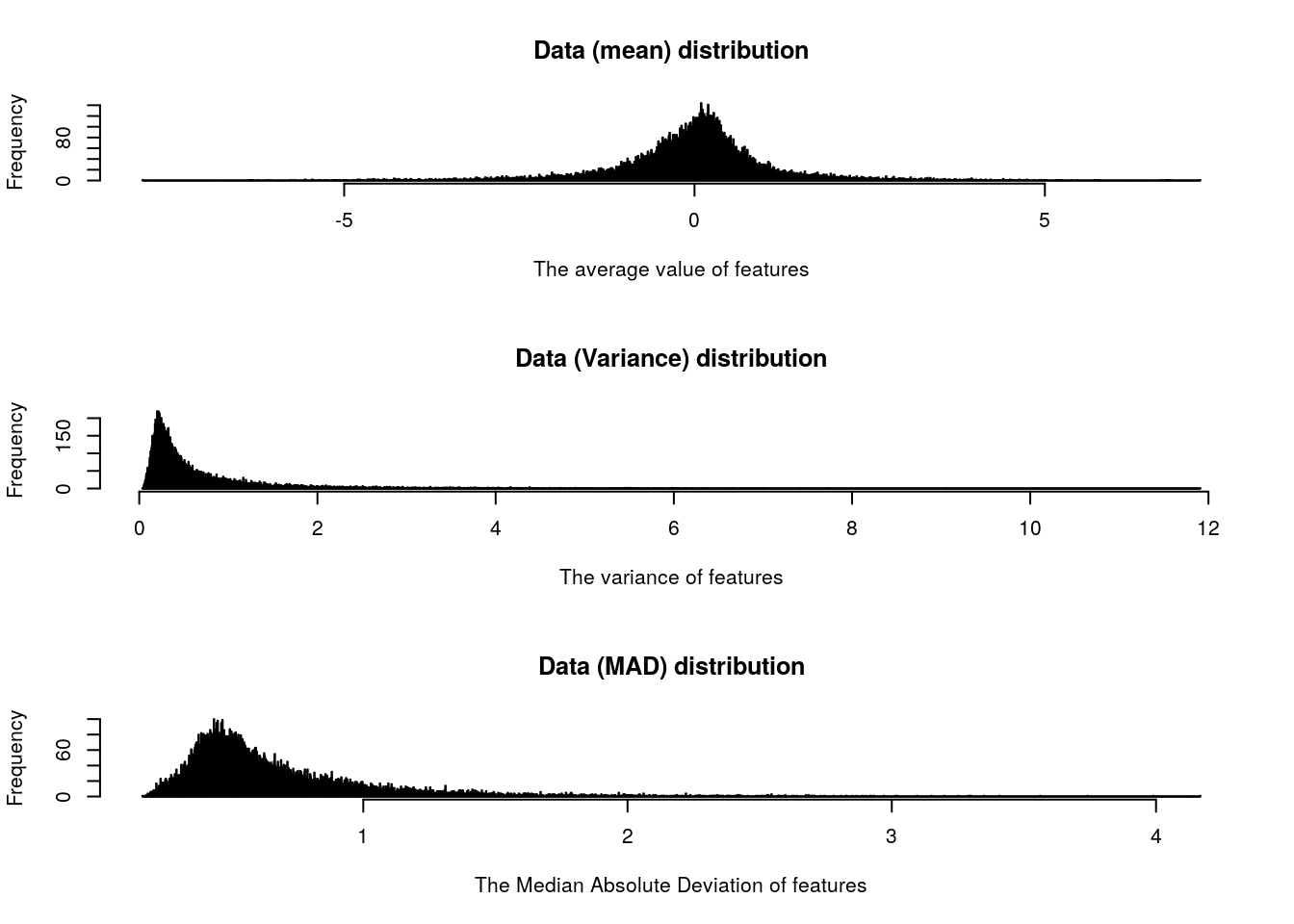#### 2.1.2 Data imputation for features with missing values (NAs)

The raw genomic dataset always contains missing observations, especially in microarray gene expression data. It is not wise to remove all the features with missing observations in very few samples because the useful information will be discarded. The common method is to impute the proper value for the missing observations. CancerSubtypes integrates three common imputation methods for genomic datasets.

``````index=which(is.na(mRNA))
res1=data.imputation(mRNA,fun="median")
res2=data.imputation(mRNA,fun="mean")
res3=data.imputation(mRNA,fun="microarray")``````

#### 2.1.3 Data normalization.

``````result1=data.normalization(mRNA,type="feature_Median",log2=FALSE)
result2=data.normalization(mRNA,type="feature_zscore",log2=FALSE)``````

### 2.2 Feature selection

#### 2.2.1 Feature selection based on the most variance.

``````###The top 1000 most variance features will be selected.
data1=FSbyVar(mRNA, cut.type="topk",value=1000)
###The features with (variance>0.5) are selected.
data2=FSbyVar(mRNA, cut.type="cutoff",value=0.5)``````

#### 2.2.2 Feature selection based on the most variant Median Absolute Deviation (MAD).

``````data1=FSbyMAD(mRNA, cut.type="topk",value=1000)

#### 2.2.3 Feature dimension reduction and extraction based on Principal Component Analysis.

``````mRNA1=data.imputation(mRNA,fun="microarray")
data1=FSbyPCA(mRNA1, PC_percent=0.9,scale = TRUE)``````

#### 2.2.4 Feature selection based on Cox regression model.

``````data(GeneExp)
data(time)
data(status)
data1=FSbyCox(GeneExp,time,status,cutoff=0.05)``````

# 3. Clustering methods for Cancer subtype identification.

### 3.1 Consensus Clustering for cancer subtype identification

Consensus clustering (CC, 2003) as an unsupervised subtypes discovery method, was a frequently used and valuable approach in many genomic studies and have lots of successful applications.

``````### The input dataset is single gene expression matrix.
data(GeneExp)
result=ExecuteCC(clusterNum=3,d=GeneExp,maxK=10,clusterAlg="hc",distance="pearson",title="GBM")

### The input dataset is multi-genomics data as a list
data(GeneExp)
data(miRNAExp)
GBM=list(GeneExp=GeneExp,miRNAExp=miRNAExp)
result=ExecuteCC(clusterNum=3,d=GBM,maxK=10,clusterAlg="hc",distance="pearson",title="GBM")``````

### 3.2 Consensus Non-negative matrix factorization for cancer subtype identification

Non-negative matrix factorization (CNMF, 2004), as an effective dimension reduction method, was used in distinguishing molecular patterns for high-dimensional genomic data and provided a powerful method for class discovery. We apply the NMF package to execute the non-negative matrix factorization for the cancer genomic dataset. So this method allows users to input the number of core-CPUs for parallel processing.

``````### The input dataset is single gene expression matrix.
data(GeneExp)
result=ExecuteCNMF(GeneExp,clusterNum=3,nrun=30)

### The input dataset is multi-genomics data as a list
data(GeneExp)
data(miRNAExp)
GBM=list(GeneExp=GeneExp,miRNAExp=miRNAExp)
result=ExecuteCNMF(GBM,clusterNum=3,nrun=30)``````

### 3.3 Integrative clustering for cancer subtype identification

Integrative clustering (iCluster, 2009) used a joint latent variable model for integrative clustering for multiple types of omics data.

``````data(GeneExp)
data(miRNAExp)
data1=FSbyVar(GeneExp, cut.type="topk",value=1000)
data2=FSbyVar(miRNAExp, cut.type="topk",value=300)
GBM=list(GeneExp=data1,miRNAExp=data2)
result=ExecuteiCluster(datasets=GBM, k=3, lambda=list(0.44,0.33,0.28))``````

### 3.4 Similarity network fusion for cancer subtype identification

Similarity network fusion (SNF, 2014) is a computational method on fusion similarity network for aggregating multi-omics data.

``````data(GeneExp)
data(miRNAExp)
GBM=list(GeneExp=GeneExp,miRNAExp=miRNAExp)
result=ExecuteSNF(GBM, clusterNum=3, K=20, alpha=0.5, t=20)``````

### 3.5 Ensemble method of SNF and CC for cancer subtype identification

We propose to combine the SNF and CC together to generate a new cancer subtypes identification method.

``````data(GeneExp)
data(miRNAExp)
data(time)
data(status)
data1=FSbyCox(GeneExp,time,status,cutoff=0.05)
data2=FSbyCox(miRNAExp,time,status,cutoff=0.05)
GBM=list(GeneExp=data1,miRNAExp=data2)
result=ExecuteSNF.CC(GBM, clusterNum=3, K=20, alpha=0.5, t=20,maxK = 10, pItem = 0.8,reps=500,
title = "GBM", plot = "png", finalLinkage ="average")``````

### 3.6 Weighted Similarity network fusion for cancer subtype identification

WSNF is a caner subtype identificaton method with the assistance of the gene regulatory network information. It makes use of the miRNA-TF-mRNA regulatory network to take the importance of the features into consideration.

``````data(GeneExp)
data(miRNAExp)
data(Ranking)
####Retrieve there feature ranking for genes
gene_Name=rownames(GeneExp)
index1=match(gene_Name,Ranking\$mRNA_TF_miRNA.v21_SYMBOL)
gene_ranking=data.frame(gene_Name,Ranking[index1,],stringsAsFactors=FALSE)
index2=which(is.na(gene_ranking\$ranking_default))
gene_ranking\$ranking_default[index2]=min(gene_ranking\$ranking_default,na.rm =TRUE)

####Retrieve there feature ranking for genes
miRNA_ID=rownames(miRNAExp)
index3=match(miRNA_ID,Ranking\$mRNA_TF_miRNA_ID)
miRNA_ranking=data.frame(miRNA_ID,Ranking[index3,],stringsAsFactors=FALSE)
index4=which(is.na(miRNA_ranking\$ranking_default))
miRNA_ranking\$ranking_default[index4]=min(miRNA_ranking\$ranking_default,na.rm =TRUE)
###Clustering
ranking1=list(gene_ranking\$ranking_default ,miRNA_ranking\$ranking_default)
GBM=list(GeneExp,miRNAExp)
result=ExecuteWSNF(datasets=GBM, feature_ranking=ranking1, beta = 0.8, clusterNum=3,
K = 20,alpha = 0.5, t = 20, plot = TRUE)``````

# 4 Results validation, interpretation and visualization for the identified cancer subtypes.

The identified cancer subtypes by the computational methods should be in accordance with biological meanings and reveal the distinct molecular patterns.

### 4.1 Silhouette width

Silhouette width is used to measure how similar a sample is matched to its identified subtype compared to other subtypes, a high value indicates that the sample is well matched. Each horizontal line represents a sample in the Silhouette plot. The length of the line is the silhouette width the sample has.

``````data(GeneExp)
data(miRNAExp)
GBM=list(GeneExp=GeneExp,miRNAExp=miRNAExp)
result=ExecuteSNF(GBM, clusterNum=3, K=20, alpha=0.5, t=20,plot = FALSE)``````
``````## Warning in SNFtool::SNF(W_temp, K = K, t = t): Dim names not consistent across all matrices in Wall.
##             Returned matrix will have no dim names.``````
``````###Similarity smaple matrix
sil=silhouette_SimilarityMatrix(result\$group, result\$distanceMatrix)
plot(sil)``````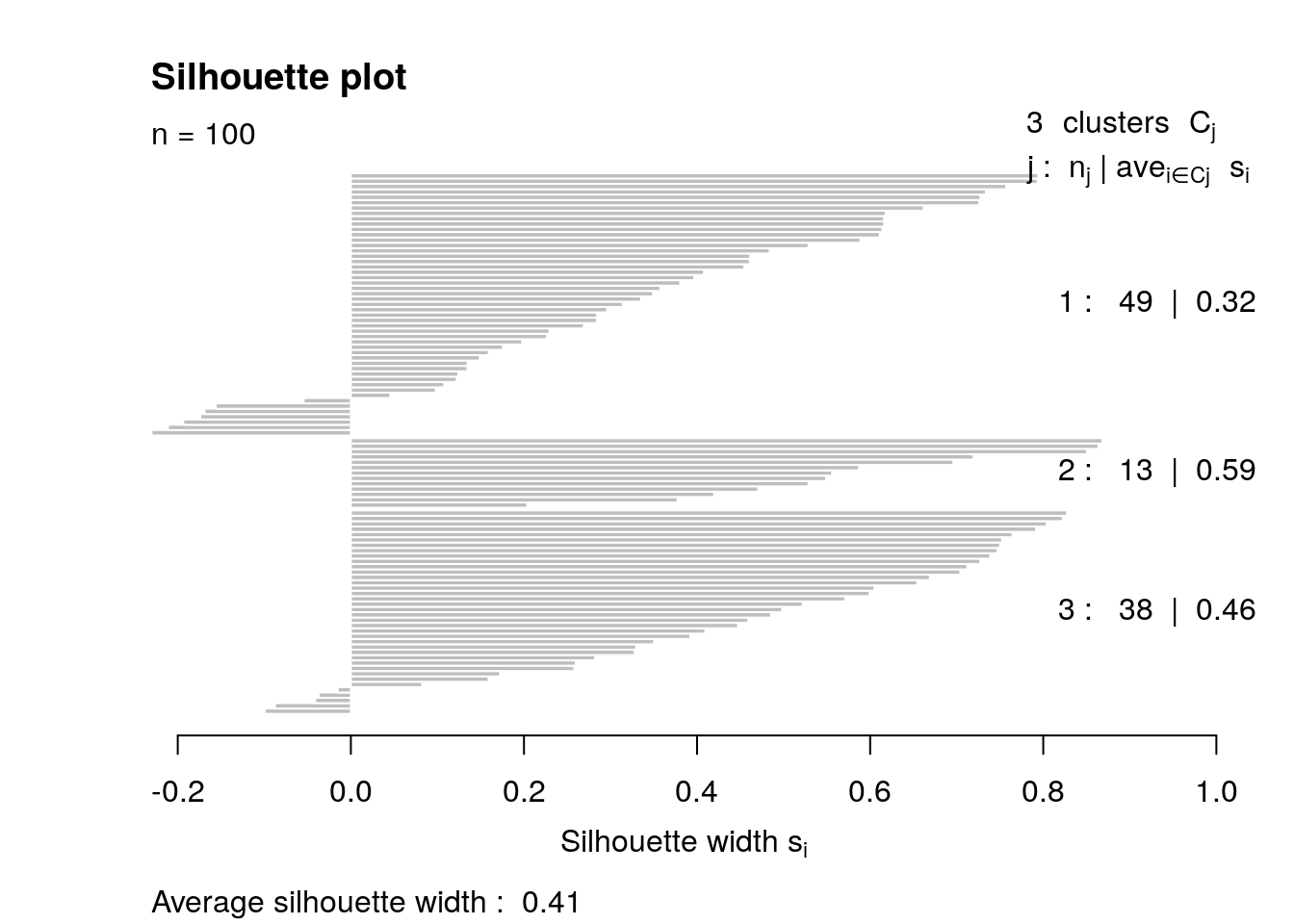Note: If the input matrix is a dissimilarity matrix between samples, please use the silhouette() in cluster package to compute the silhouette width, otherwise a wrong result will be generated.

``````sil1=silhouette(result\$group, result\$distanceMatrix)
plot(sil1)  ##wrong result``````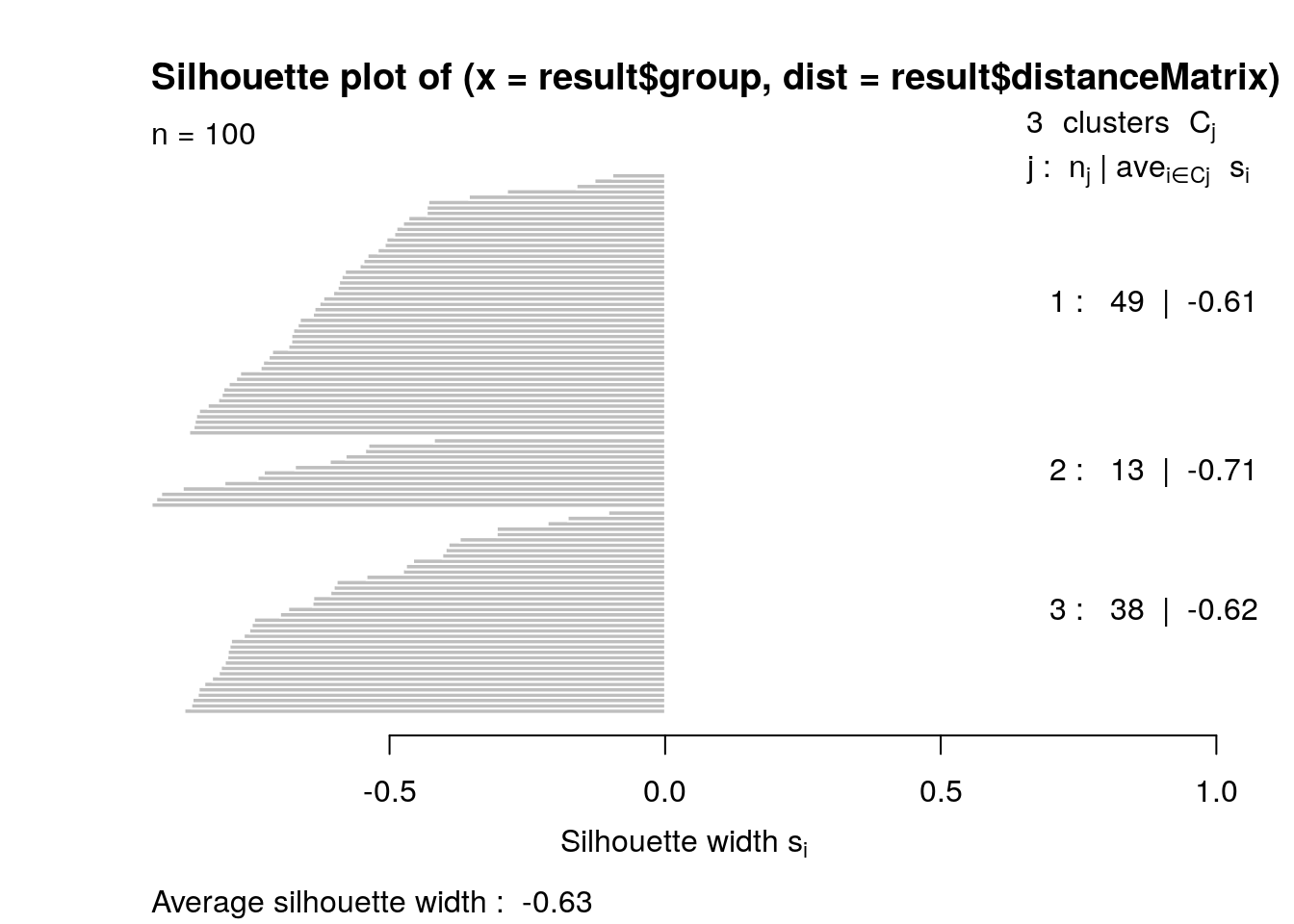All the samples have the negative silhouette width.

### 4.2 Survival analysis

Survival analysis is used to judge the different survival patterns between subtypes.

``````data(GeneExp)
data(miRNAExp)
data(time)
data(status)
data1=FSbyCox(GeneExp,time,status,cutoff=0.05)
data2=FSbyCox(miRNAExp,time,status,cutoff=0.05)
GBM=list(GeneExp=data1,miRNAExp=data2)

#### 1.ExecuteSNF
result1=ExecuteSNF(GBM, clusterNum=3, K=20, alpha=0.5, t=20,plot = FALSE)``````
``````## Warning in SNFtool::SNF(W_temp, K = K, t = t): Dim names not consistent across all matrices in Wall.
##             Returned matrix will have no dim names.``````
``````group1=result1\$group
distanceMatrix1=result1\$distanceMatrix
p_value=survAnalysis(mainTitle="GBM1",time,status,group1,
distanceMatrix1,similarity=TRUE)``````
``````##
## *****************************************************
## GBM1 Cluster= 3   Call:
## survdiff(formula = Surv(time, status) ~ group)
##
##          N Observed Expected (O-E)^2/E (O-E)^2/V
## group=1 46       44     41.3     0.173     0.311
## group=2 40       39     27.3     4.990     7.387
## group=3 14       13     27.4     7.529    11.661
##
##  Chisq= 14.2  on 2 degrees of freedom, p= 8e-04``````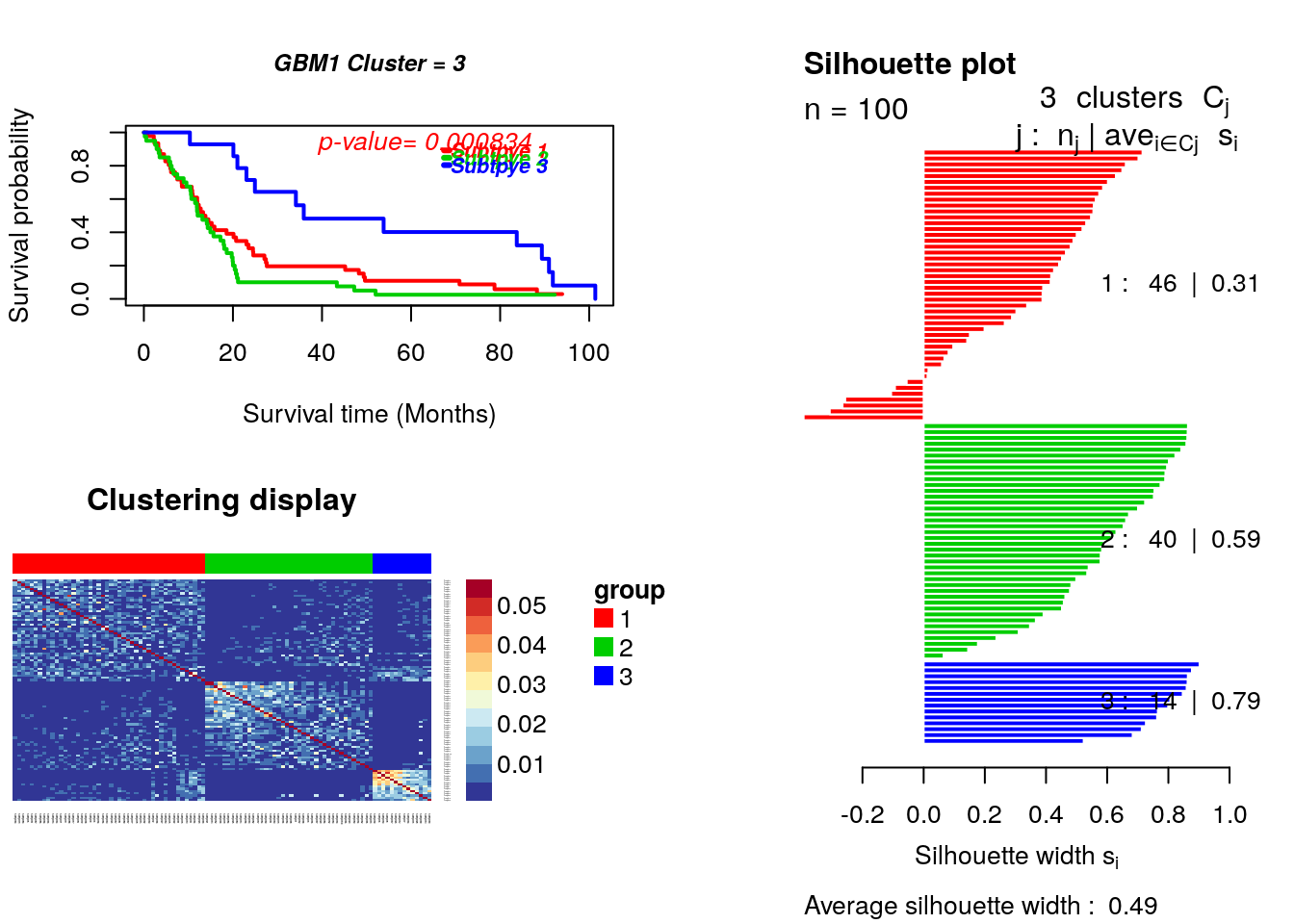This is a combination figure with three parts: Survival curves, heatmap of the sample similarity matrix and Silhouette width plots for the identified cancer subtypes. The samples in all of the plots have be reorganized by the identified caner subtypes. This kind of figure provides the visible results that can be easily evaluated.

``````#### 2.ExecuteSNF.CC
result2=ExecuteSNF.CC(GBM, clusterNum=3, K=20, alpha=0.5, t=20,
maxK = 5, pItem = 0.8,reps=500,
title = "GBM2", plot = "png",
``````## Warning in SNFtool::SNF(W_temp, K = K, t = t): Dim names not consistent across all matrices in Wall.
##             Returned matrix will have no dim names.``````
``````group2=result2\$group
distanceMatrix2=result2\$distanceMatrix
p_value=survAnalysis(mainTitle="GBM2",time,status,group2,
distanceMatrix2,similarity=TRUE)``````
``````##
## *****************************************************
## GBM2 Cluster= 3   Call:
## survdiff(formula = Surv(time, status) ~ group)
##
##          N Observed Expected (O-E)^2/E (O-E)^2/V
## group=1 40       39     27.3     4.990     7.387
## group=2 46       44     41.3     0.173     0.311
## group=3 14       13     27.4     7.529    11.661
##
##  Chisq= 14.2  on 2 degrees of freedom, p= 8e-04``````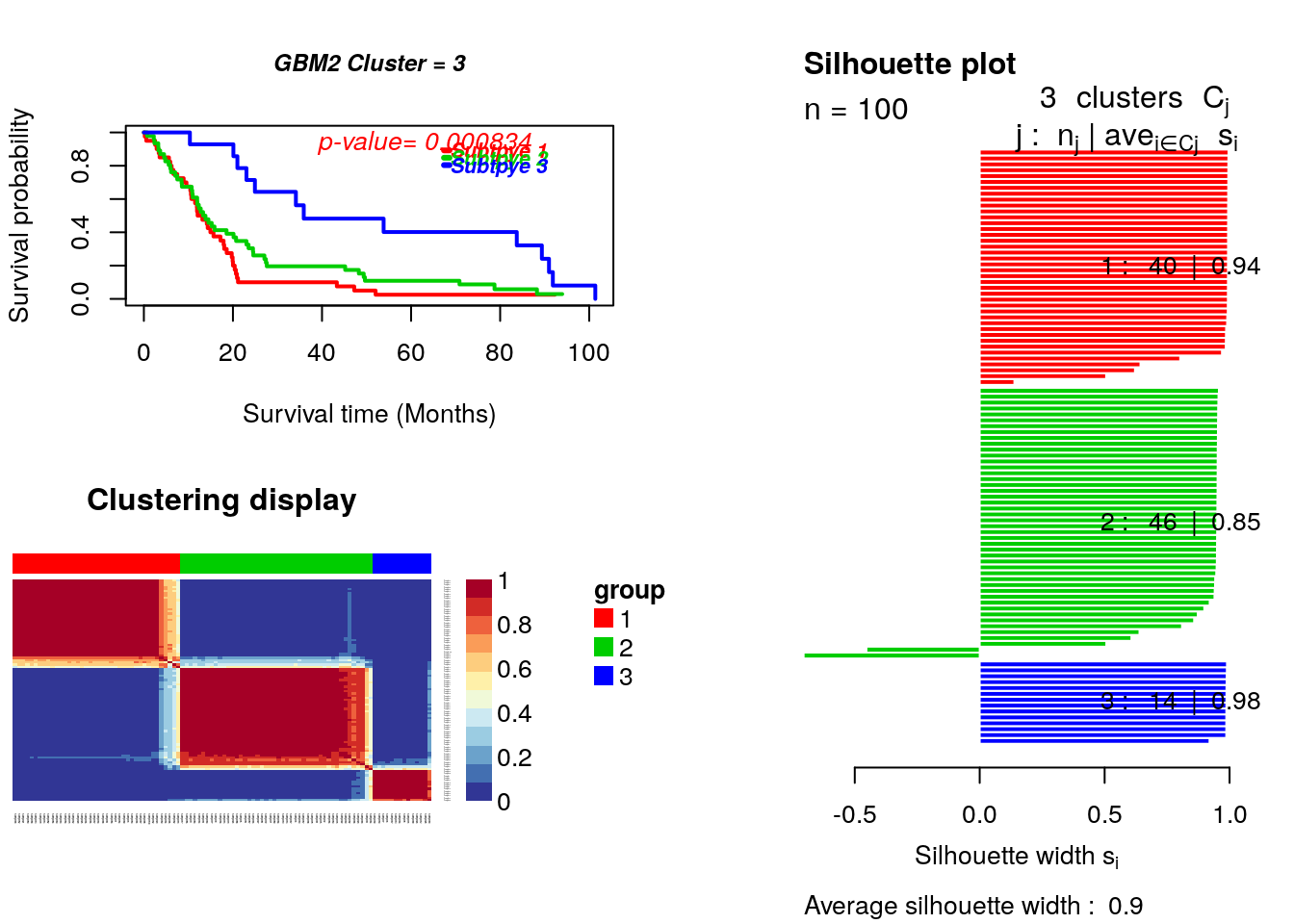### 4.3 Statistical significance of clustering

Statistical significance of clustering is a pure statistical approach to test the significance difference data distribution between subtypes. Different expression is to test the expression difference between each subtypes and a reference group (always a set of normal samples).

``````data(GeneExp)
data(miRNAExp)
data(time)
data(status)
GBM=list(GeneExp=GeneExp,miRNAExp=miRNAExp)
result=ExecuteSNF(GBM, clusterNum=3, K=20, alpha=0.5, t=20,plot = FALSE)
group=result\$group
sigclust=sigclustTest(miRNAExp,group, nsim=1000, nrep=1, icovest=1)``````